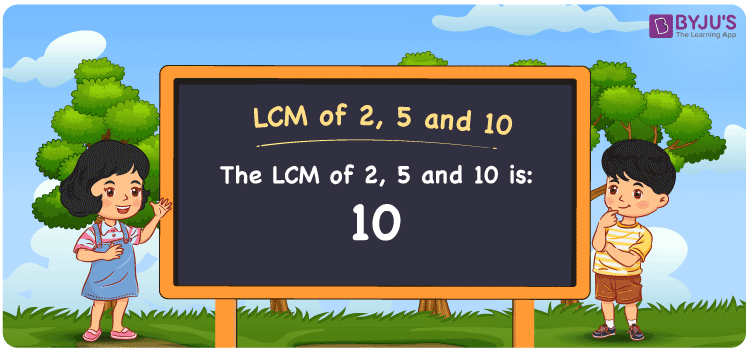Checkout JEE MAINS 2022 Question Paper Analysis : Checkout JEE MAINS 2022 Question Paper Analysis :

# LCM of 2, 5 and 10

LCM of 2, 5 and 10 is 10. The number which is evenly divisible by the two given numbers determines the LCM. By listing out the multiples, you will know how to identify the common multiple which is the LCM. The multiples of 2 are (2, 4, 6, 8, 10, ….), the multiples of 5 are (5, 10, 15, 20, 25, …. ) and the multiples of 10 are (10, 20, 30, 40, 50, 60, ….) respectively. The procedures followed to calculate the LCM are division, prime factorization and listing the multiples.

## What is LCM of 2, 5 and 10?

The answer to this question is 10. The LCM of 2, 5 and 10 using various methods is shown in this article for your reference. The LCM of two non-zero integers, 2, 5 and 10, is the smallest positive integer 10 which is divisible by both 2, 5 and 10 with no remainder.## How to Find LCM of 2, 5 and 10?

LCM of 2, 5 and 10 can be found using three methods:

• Prime Factorisation
• Division method
• Listing the multiples

### LCM of 2, 5 and 10 Using Prime Factorisation Method

The prime factorisation of 2, 5 and 10, respectively, is given by:

2 = 2¹

5 = 5¹

10 = 2 x 5 = 2¹ x 5¹

LCM (2, 5, 10) = 10

### LCM of 2, 5 and 10 Using Division Method

We’ll divide the numbers (2, 5, 10) by their prime factors to get the LCM of 2, 5 and 10 using the division method (preferably common). The LCM of 2, 5 and 10 is calculated by multiplying these divisors.

 2 2 5 10 5 1 5 5 x 1 1 1

No further division can be done.

Hence, LCM (2, 5, 10) = 10

### LCM of 2, 5 and 10 Using Listing the Multiples

To calculate the LCM of 2, 5 and 10 by listing out the common multiples, list the multiples as shown below

 Multiples of 2 Multiples of 5 Multiples of 10 2 5 10 4 10 20 6 15 30 8 20 40 10 25 50 12 30 60

LCM (2, 5, 10) = 10

## Video Lesson on Applications of LCM## LCM of 2, 5 and 10 Solved Examples

What is the smallest number that is exactly divisible by 2, 5 and 10?

Solution:

LCM is the smallest number exactly divisible by 2, 5 and 10

Multiples of 2 = 2, 4, 6, 8, 10, ….

Multiples of 5 = 5, 10, 15, 20, 25, ….

Multiples of 10 = 10, 20, 30, 40, 50, …..

Hence, the LCM of 2, 5 and 10 is 10.

## Frequently Asked Questions on LCM of 2, 5 and 10

### What is the LCM of 2, 5 and 10?

The LCM of 2, 5 and 10 is 10.

### What are the methods used to find the LCM of 2, 5 and 10?

The methods used to find the LCM of 2, 5 and 10 are prime factorisation, division method and listing multiples.

### Write the relation between GCF and LCM of 2, 5 and 10.

The relation between GCF and LCM of 2, 5 and 10 are

LCM x GCF = 2 x 5 x 10

LCM x GCF = 100